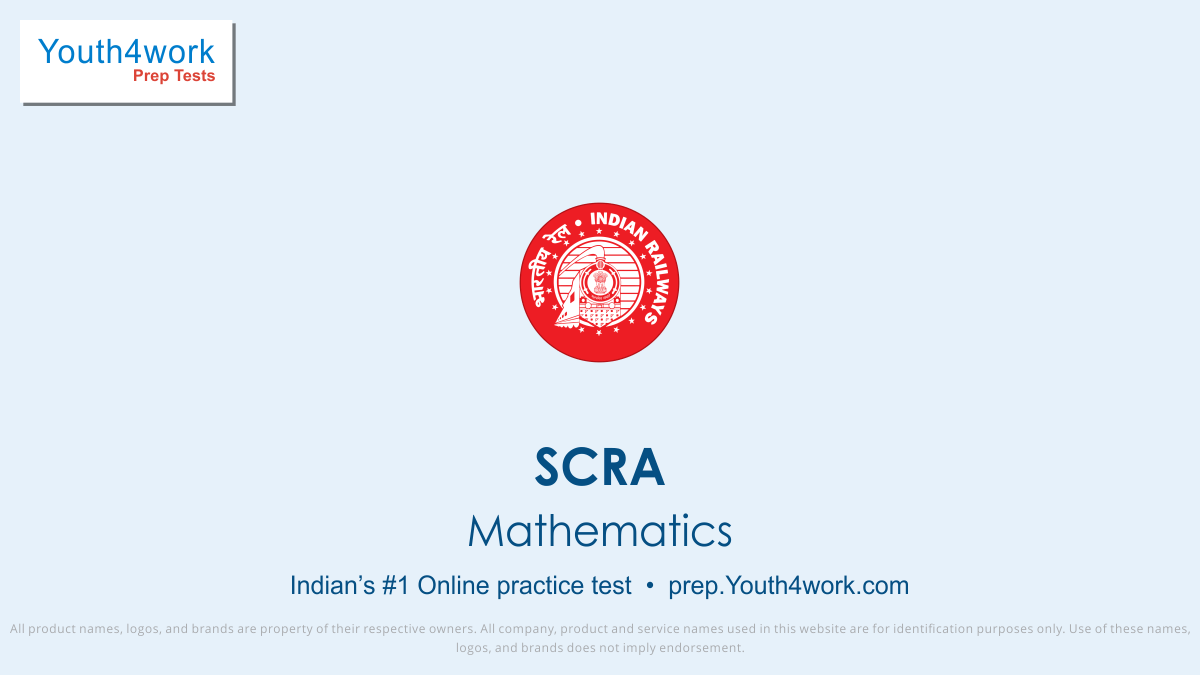# Examen online de práctica para Mathematics Scra

SCRA Vector Mock Test will challenge you and compel you towards using mathematical logic instead of crammed up shortcuts. Apply logic while solving Mathematics questions on the topic Vector and Its Applications.

The questions asked in SCRA Probability Mock Test will be designed to test the candidates' understanding and command of the Elementary theorems on probability simple problems. Begin the test and see for yourself.

Try as many SCRA Geometry Mock Test as possible to understand the concept of geometry. Geometry Tests for Special Class Railway Apprentice (SCRA) Exam tests your skill in visualizing figures and analyze the questions.

This SCRA Trigonometry Mock Test deals with the problems of Trigonometry. Trigonometry is one of the most important topics in SCRA Exam which can be improved by regularly attempting SCRA Practice Test.

Youth4Work's SCRA Algebra Mock Test will compel you to use your brain's maximum capacity so you may ace the exam. The Algebra asked in SCRA Exam is designed to assess a candidate's ability to work with equations and variables.